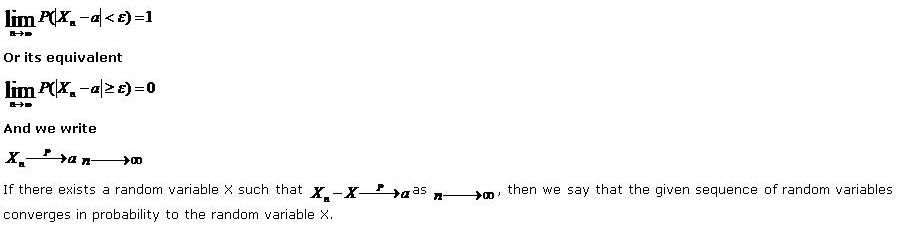# Statistics Assignment Help With Convergence In Probability

## 4.13. Convergence In Probability:

We shall now introduce a new concept of convergence, that is convergence in probability or stochastic convergence which is defined as follows

A sequence of random variables X1, X2,…. Xn,… is said to converge in probability to a constant a, if for any Є >0.

###